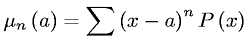Equations > Statistics > Moments > nth moment about point a

### nth moment about point aLatex Code:

MathML Code:

 ${\mu }_{n}\left(a\right)=\sum {\left(x-a\right)}^{n}P\left(x\right)$

MathType 5.0: